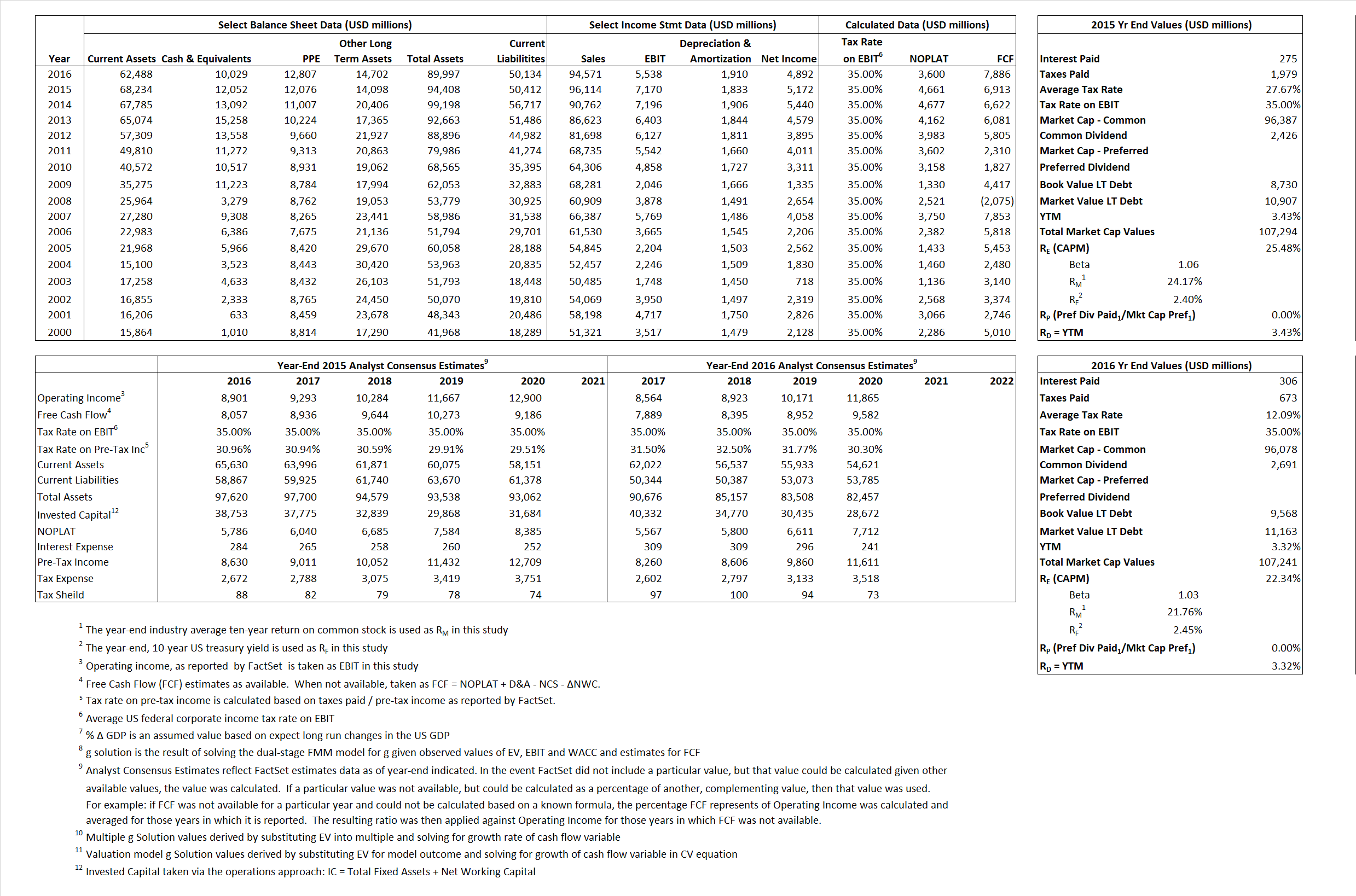# Boeing

## Analyst Listing

The following analysts provide coverage for the subject firm as of May 2016:

The following analysts provide coverage for the subject firm as of May 2016:

 Broker Analyst Analyst Email Cowen & Company Cai von Rumohr cai.von-rumohr@cowen.com Bernstein Research Douglas S. Harned douglas.harned@bernstein.com Jefferies Howard A. Rubel hrubel@jefferies.com Wolfe Research Hunter K. Keay hkeay@wolferesearch.com Canaccord Genuity Ken Herbert kherbert@canaccordgenuity.com Deutsche Bank Research Myles Walton myles.walton@db.com CRT Capital Group Peter Arment parment@sterneageecrt.com Drexel Hamilton Peter Skibitski pskibitski@drexelhamilton.com Buckingham Research Richard Safran rsafran@buckresearch.com Credit Suisse Robert Spingarn robert.spingarn@credit-suisse.com RBC Capital Markets Robert Stallard robert.stallard@rbccm.com Wells Fargo Securities Sam J. Pearlstein sam.pearlstein@wellsfargo.com

## Primary Input Data## Derived Input Data

### Equational Form

Net Operating Profit Less Adjusted Taxes NOPLAT 4,661 3,600$NOPLAT\, =\, EBIT\, x\, (1 \,-\, Avg \,\,Tax\,\, Rate\,\, on\,\, EBIT)$
Free Cash Flow FCF 6,913 7,886$FCF\,=NOPLAT\,+\,Non-Cash\,Expenses-\Delta NWC\,-\,NCS$
Tax Shield TS 76 37$TS\,=\,Interest\,\,Paid\,\,x\,\, Avg \,\,Tax\,\,Rate\,\, on\,\, Pre-Tax\,\, Income$
Invested Capital IC 43,996 39,863$IC\,=\,Fixed\,\,Operating\,\,Assets\,\,+\,\,Net\,\, Working\,\, Capital$
Return on Invested Capital ROIC 10.59% 9.03%$ROIC\,=\,\frac { NOPLAT }{ IC }$
Net Investment NetInv 3,348 (2,223)$NetInv\,=\,{ {IC}_{1}}-{{IC}_{0}}+Depreciation$
Investment Rate IR  71.84% -61.76%$IR\,=\,\frac {NetInv}{NOPLAT}$
Weighted Average Cost of Capital
WACCMarket  23.14% 20.32%$WACC\,=\,\frac { E }{ V } { R }_{ E }\,+\,\frac { P }{ V } { R }_{ P }\,+\,\frac { D }{ V } { R }_{ D }\left( 1- Avg\,\, Tax\,\,Rate\,\,on\,\,Pre-Tax\,\,Income \right)$
WACCBook   9.07%  8.54%
Enterprise value
EVMarket  95,242 97,212$EV\,=\,Market\,\,Cap\,\,Equity\,+\,\,Long\,\,Term\,\,Debt\,-\,Cash$
EVBook  93,065  95,617
Long-Run Growth
g = IR x ROIC
7.61%  -5.58% Long-run growth rates of the income variable are used in the Continuing Value portion of the valuation models.
g = %$\Delta$ GDP   2.50%  2.50%
Margin from Operations M   7.46%  5.86%$M\,\,=\,\,\frac{EBIT}{SALES}$
Depreciation/Amortization Rate D  20.36%  25.64%$D\,\,=\,\,\frac{D+A}{EBITDA}$

## Valuation Multiple Outcomes

The outcomes presented in this study are the result of original input data, derived data, and synthesized inputs.

### model g solution

12/31/2015 12/31/2016 12/31/2015 12/31/2016 12/31/2015 12/31/2016

EV/SALES$\frac {EV}{Sales} \,= \,\frac{ROIC\, -\, g}{ROIC\,(WACC\,-\,g)}\,(1\,-\,T)\,(M)$

.99 1.03  33.91%  28.16%  26.72%  23.06%

EV/EBITDA$\frac {EV}{EBITDA} \,= \,\frac{ROIC\, -\, g}{ROIC\,(WACC\,-\,g)}\,(1\,-\,T)\,(1\,-\,D)$

10.58 13.05 33.91% 28.16% 26.72% 23.06%

EV/NOPLAT$\frac {EV}{NOPLAT} \,= \,\frac{ROIC\, -\, g}{ROIC\,(WACC\,-\,g)}$

20.44 27.01 33.91% 28.16% 26.72% 23.06%

EV/FCFOPS$\frac {EV}{FCF_{OPS}} \,= \,\frac{ROIC\, -\, g}{ROIC\,(WACC\,-\,g)}\,(1\,-\,T)$

13.78 12.33 33.91% 28.16% 26.72% 23.06%

EV/EBIT$\frac {EV}{EBIT} \,= \,\frac{ROIC\, -\, g}{ROIC\,(WACC\,-\,g)}\,(1\,-\,T)$

13.28 17.55 33.91% 28.16% 26.72% 23.06%

EV/IC$\frac {EV}{IC} \,= \,\frac{ROIC\, -\, g}{WACC\,-\,g}$

2.16 2.44 33.91% 28.16% 26.72% 23.06%